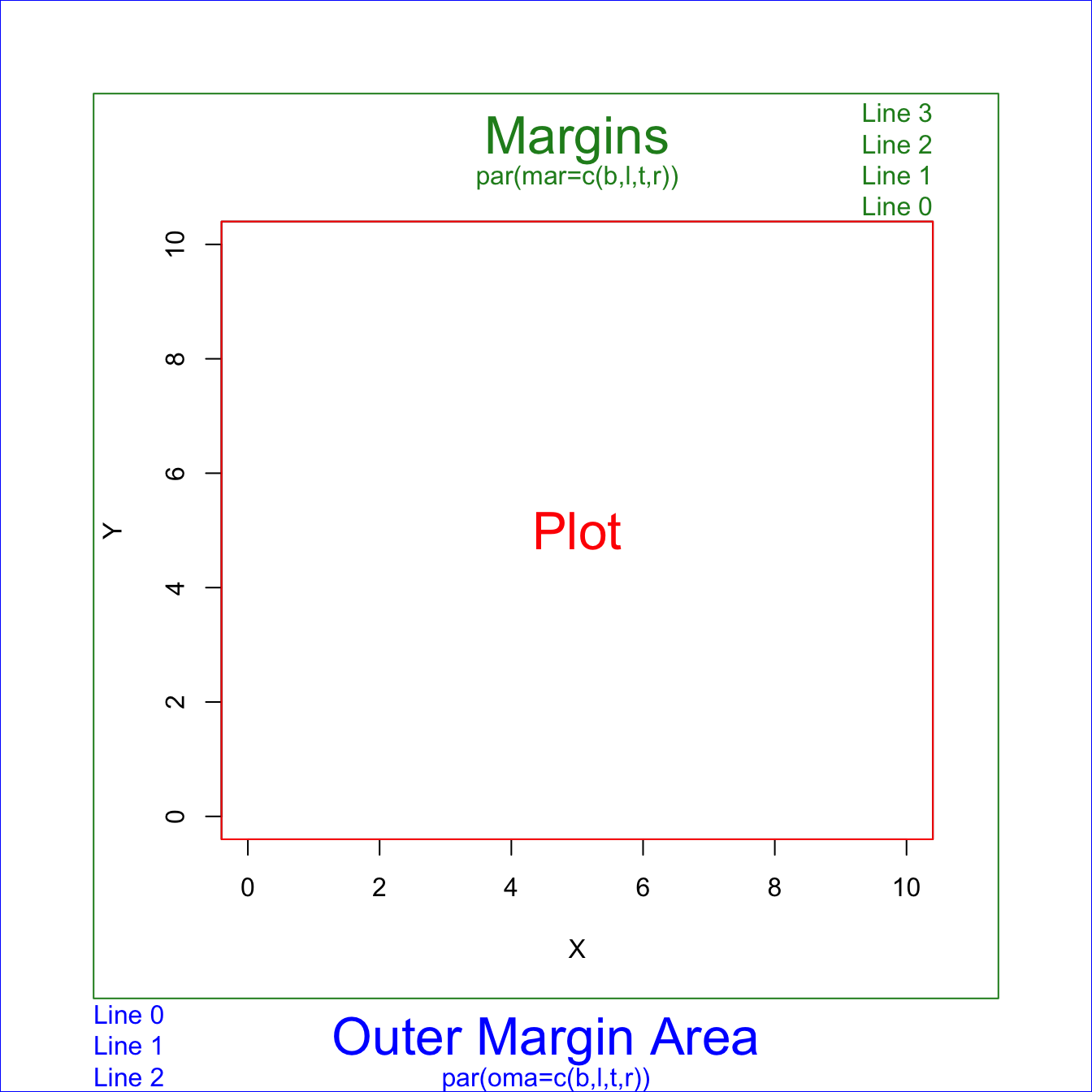# Base R margins: a cheatsheet.

This post is dedicated to margin management in `base R`. It describes how to use the `par` function to call the `mar` and `oma` parameters: the 2 types of margin.

There are 2 margin areas in `base R` plots: `margin` and `oma`. You can control their size calling the `par()` function before your plot and giving the corresponding arguments:

• `mar()` for margin.
• `oma()` for outer margin area.

For both arguments, you must give four values giving the desired space in the bottom, left, top and right part of the chart respectively. For instance, `par(mar=c(4,0,0,0))` draws a margin of size 4 only on the bottom of the chart.

You can use `mai()` and `omi()` if you want to set the areas in inches and not in lines.``````# Margins area
par(oma=c(3,3,3,3)) # all sides have 3 lines of space
par(mar=c(5,4,4,2) + 0.1)

# Plot
plot(0:10, 0:10, type="n", xlab="X", ylab="Y") # type="n" hides the points

# Place text in the plot and color everything plot-related red
text(5,5, "Plot", col="red", cex=2)
box(col="red")

# Place text in the margins and label the margins, all in forestgreen
mtext("Margins", side=3, line=2, cex=2, col="forestgreen")
mtext("par(mar=c(b,l,t,r))", side=3, line=1, cex=1, col="forestgreen")
mtext("Line 0", side=3, line=0, adj=1.0, cex=1, col="forestgreen")
mtext("Line 1", side=3, line=1, adj=1.0, cex=1, col="forestgreen")
mtext("Line 2", side=3, line=2, adj=1.0, cex=1, col="forestgreen")
mtext("Line 3", side=3, line=3, adj=1.0, cex=1, col="forestgreen")
box("figure", col="forestgreen")

# Label the outer margin area and color it blue
# Note the 'outer=TRUE' command moves us from the figure margins to the outer margins.
mtext("Outer Margin Area", side=1, line=1, cex=2, col="blue", outer=TRUE)
mtext("par(oma=c(b,l,t,r))", side=1, line=2, cex=1, col="blue", outer=TRUE)
mtext("Line 0", side=1, line=0, adj=0.0, cex=1, col="blue", outer=TRUE)
mtext("Line 1", side=1, line=1, adj=0.0, cex=1, col="blue", outer=TRUE)
mtext("Line 2", side=1, line=2, adj=0.0, cex=1, col="blue", outer=TRUE)
box("outer", col="blue")  ``````

Related chart types

## Contact

This document is a work by Yan Holtz. Any feedback is highly encouraged. You can fill an issue on Github, drop me a message on Twitter, or send an email pasting yan.holtz.data with gmail.com.